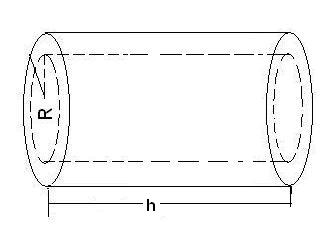# Pipe Volume Calculator

Calculate the cylindrical volume of a tube for given values.

 Cylindrical Volume

Calculate the cylindrical volume of a tube, also called a pipe or hollow cylinder for given values using :

### Formula

cylindrical volume (V) = π(r12 - r22 )h

where,